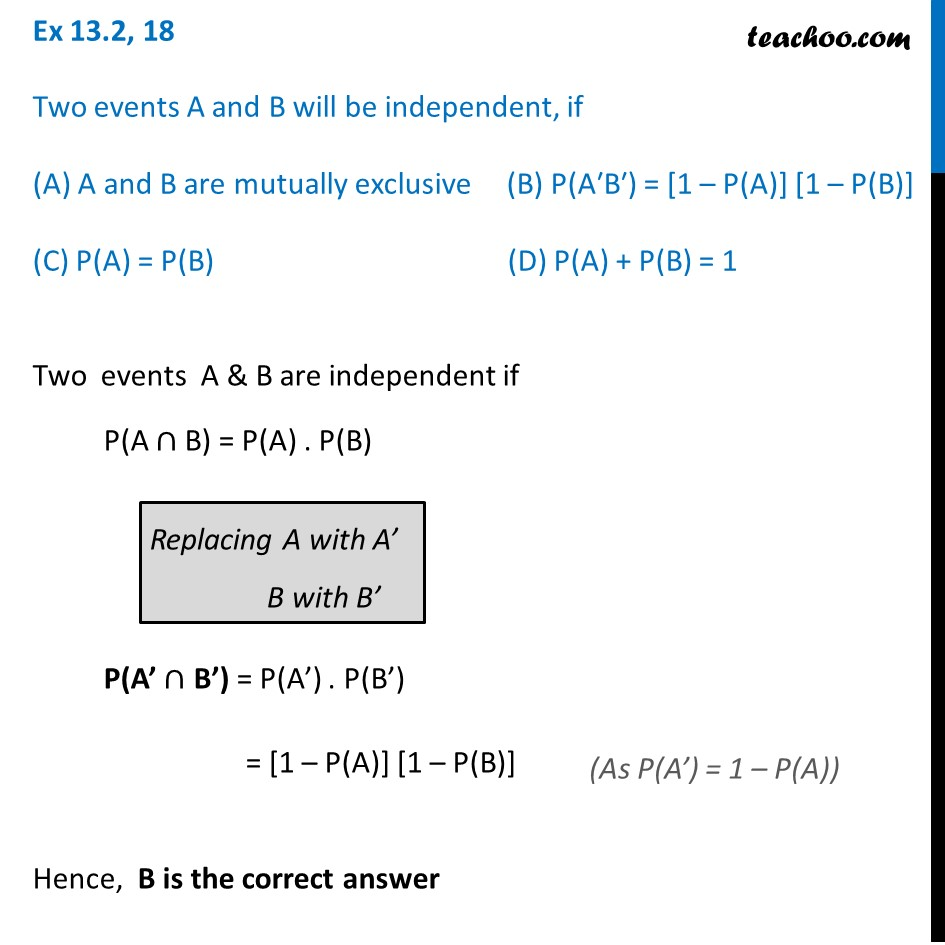Ex 13.2

Chapter 13 Class 12 Probability
Serial order wiseLearn in your speed, with individual attention - Teachoo Maths 1-on-1 Class

### Transcript

Ex 13.2, 18 Two events A and B will be independent, if (A) A and B are mutually exclusive (B) P(A′B′) = [1 – P(A)] [1 – P(B)] (C) P(A) = P(B) (D) P(A) + P(B) = 1Two events A & B are independent if P(A ∩ B) = P(A) . P(B) Replacing A with A’ B with B’ P(A’ ∩ B’) = P(A’) . P(B’) = [1 – P(A)] [1 – P(B)] Hence, B is the correct answer